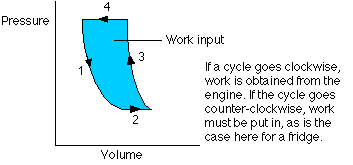## Thermodynamics

6-25-98

### The second law of thermodynamics

The second law of thermodynamics comes in more than one form, but let's state in a way that makes it obviously true, based on what you've observed from simply being alive.

The second law states that heat flows naturally from regions of higher temperature to regions of lower temperature, but that it will not flow naturally the other way.

Heat can be made to flow from a colder region to a hotter region, which is exactly what happens in an air conditioner, but heat only does this when it is forced. On the other hand, heat flows from hot to cold spontaneously.

The second law of thermodynamics is one of the most fundamental laws of nature, having profound implications. In essence, it says this:

The second law - The level of disorder in the universe is steadily increasing. Systems tend to move from ordered behavior to more random behavior.

One implication of the second law is that heat flows spontaneously from a hotter region to a cooler region, but will not flow spontaneously the other way. This applies to anything that flows: it will naturally flow downhill rather than uphill.

If you watched a film forwards and backwards, you would almost certainly be able to tell which way was which because of the way things happen. A pendulum will gradually lose energy and come to a stop, but it doesn't pick up energy spontaneously; an ice cube melts to form a puddle, but a puddle never spontaneously transforms itself into an ice cube; a glass falling off a table might shatter when it hits the ground, but the pieces will never spontaneously jump back together to form the glass again. Many processes are irreversible, and any irreversible process increases the level of disorder. One of the most important implications of the second law is that it indicates which way time goes - time naturally flows in a way that increases disorder.

The second law also predicts the end of the universe: it implies that the universe will end in a "heat death" in which everything is at the same temperature. This is the ultimate level of disorder; if everything is at the same temperature, no work can be done, and all the energy will end up as the random motion of atoms and molecules.

### Entropy

A measure of the level of disorder of a system is entropy, represented by S. Although it's difficult to measure the total entropy of a system, it's generally fairly easy to measure changes in entropy. For a thermodynamic system involved in a heat transfer of size Q at a temperature T , a change in entropy can be measured by: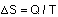The second law of thermodynamics can be stated in terms of entropy. If a reversible process occurs, there is no net change in entropy. In an irreversible process, entropy always increases, so the change in entropy is positive. The total entropy of the universe is continually increasing.

There is a strong connection between probability and entropy. This applies to thermodynamic systems like a gas in a box as well as to tossing coins. If you have four pennies, for example, the likelihood that all four will land heads up is relatively small. It is six times more likely that you'll get two heads and two tails. The two heads - two tails state is the most likely, shows the most disorder, and has the highest entropy. Four heads is less likely, has the most order, and the lowest entropy. If you tossed more coins, it would be even less likely that they'd all land heads up, and even more likely that you'd end up with close to the same number of heads as tails.

With a gas in a box, the probability that all the gas molecules are in one corner of the box at the same time is very small (for a typical box full of 1020 molecules or more, incredibly small): this is therefore a low entropy state. It is much more likely that the molecules are randomly distributed around the box, and are moving in random directions; this high disorder state is a considerably higher entropy state. The second law doesn't rule out all the molecules ending up in one corner, but it means it's far more likely that the molecules will be randomly distributed, and to move towards a random distribution from an orderly distribution, as opposed to the other way around.

### Heat engines

We'll move on to look at heat engines, which are devices that use heat to do work. A basic heat engine consists of a gas confined by a piston in a cylinder. If the gas is heated, it expands, moving the piston. This wouldn't be a particularly practical engine, though, because once the gas reaches equilibrium the motion would stop. A practical engine goes through cycles; the piston has to move back and forth. Once the gas is heated, moving the piston up, it can be cooled and the piston will move back down. A cycle of heating and cooling will move the piston up and down.

A necessary component of a heat engine, then, is that two temperatures are involved. At one stage the system is heated, at another it is cooled.

In a full cycle of a heat engine, three things happen:

1. Heat is added. This is at a relatively high temperature, so the heat can be called QH.
2. Some of the energy from that input heat is used to perform work (W).
3. The rest of the heat is removed at a relatively cold temperature (QC).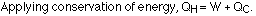The following diagram is a representation of a heat engine, showing the energy flow: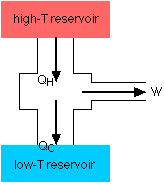An important measure of a heat engine is its efficiency: how much of the input energy ends up doing useful work? The efficiency is calculated as a fraction (although it is often stated as a percentage):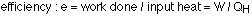Work is just the input heat minus the rejected heat, so: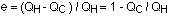Note that this is the maximum possible efficiency for an engine. In reality there will be other losses (to friction, for example) that will reduce the efficiency.

### Carnot's principle

How can an engine achieve its maximum efficiency? It must operate using reversible processes: a reversible process is one in which the system and the surroundings can be returned to state they were in before the process began. If energy is lost to friction during a process, the process is irreversible; if energy is lost as heat flows from a hot region to a cooler region, the process is irreversible. The efficiency of an engine using irreversible processes can not be greater than the efficiency of an engine using reversible processes that is working between the same temperatures. This is known as Carnot's principle, named after Sadi Carnot, a French engineer.

For any reversible engine (known as a Carnot engine) operating between two temperatures, TH and TC, the efficiency is given by:The efficiency is maximized when the cold reservoir is as cold as possible, and the hot temperature is as hot as possible.

### The third law

The third law of thermodynamics states this : it is impossible to reach absolute zero. This implies that a perpetual motion machine is impossible, because the efficiency will always be less than 1.

### Refrigerators, air conditioners, etc.

A device such as a refrigerator or air conditioner, designed to remove heat from a cold region and transfer it to a hot region, is essentially a heat engine operating in reverse, as the following energy flow diagram shows: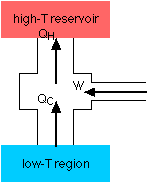A refrigerator, consisting of a fluid pumped through a closed system, involves a four-step process. An air conditioner works the same way.

• Step 1 - The fluid passes through a nozzle and expands into a low-pressure area. Similar to the way carbon dioxide comes out of a fire extinguisher and cools down, the fluid turns into a gas and cools down. This is essentially an adiabatic expansion.
• Step 2 - The cool gas is in thermal contact with the inner compartment of the fridge; it heats up as heat is transferred to it from the fridge. This takes place at constant pressure, so it's an isobaric expansion.
• Step 3 - The gas is transferred to a compressor, which does most of the work in this process. The gas is compressed adiabatically, heating it and turning it back to a liquid.
• Step 4 - The hot liquid passes through coils on the outside of the fridge, and heat is transferred to the room. This is an isobaric compression process.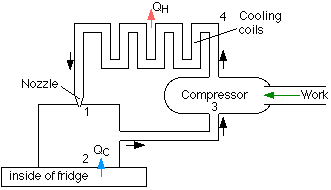A refrigerator is rated by something known as the coefficient of performance, which is the ratio of the heat removed from the fridge to the work required to remove it: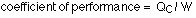### The P-V graph for a refrigerator cycle

The P-V (pressure-volume) graph is very useful for calculating the work done. For any kind of heat engine or refrigerator (reverse heat engine), the processes involved form a cycle on the P-V graph. The work is the area of the enclosed region on the graph. The diagram for a refrigerator is a little more complicated than this because of the two phase changes involved, but this is basically what it looks like: